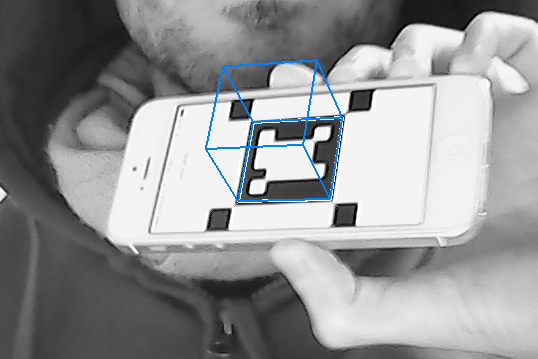I am sure some people are hitting their heads trying to solve this simple problem when doing augmented reality with OpenCV and OpenGL:

How to get the proper OpenGL projection matrix from the OpenCV camera calibration values?

For the simple common case where the OpenCV camera matrix has the form:

``````|fx  0 cx|
|0  fy cy|
|0  0   1|
``````

The corresponding OpenGL projection matrix can be computed like this:

``````m = 2.0 * fx / width;
m = 0.0;
m = 0.0;
m = 0.0;

m = 0.0;
m = -2.0 * fy / height;
m = 0.0;
m = 0.0;

m = 1.0 - 2.0 * cx / width;
m = 2.0 * cy / height - 1.0;
m = (zfar + znear) / (znear - zfar);
m = -1.0;

m = 0.0;
m = 0.0;
m = 2.0 * zfar * znear / (znear - zfar);
m = 0.0;
``````

Where `height` and `width` are the size of the captured image ; `znear` and `zfar` are the clipping value for the projection.

It took me a lot of time to get it right, since we have to be careful of the difference in referential between OpenGL and OpenCV.